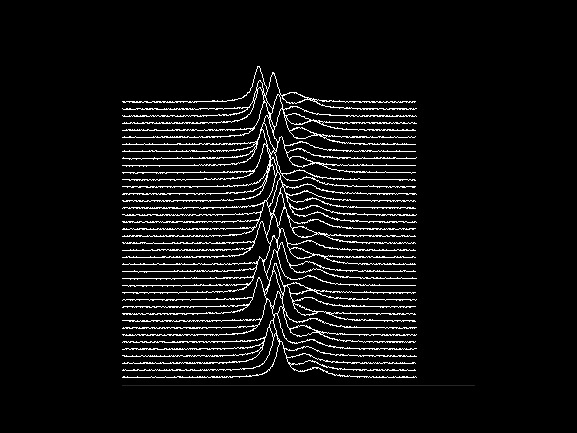• /
• # Joy Division

on 11 Oct 2021
• 11
• 105
• 1
• 0
• 226
% Creates a ridgeline plot similar to the "Unknown Pleasures" album cover
% by Joy Division
y=humps(-2:0.01:3);
y=y-min(y);
y=repmat(y,[40 1])+(800:-20:20)';
for i=1:40
y(i,:)=circshift(y(i,:),randi(50,1))+3*rand(1,length(y(i,:)));
area(y(i,:),FaceColor='k')
hold on
h=plot(y(i,:),Color='w');
end
set(gcf,'color','k')
axis square off